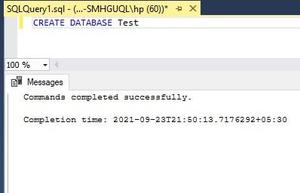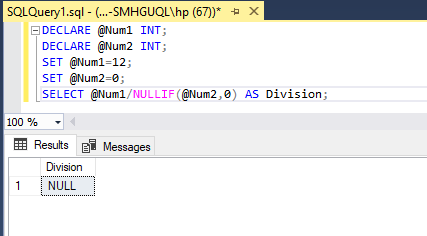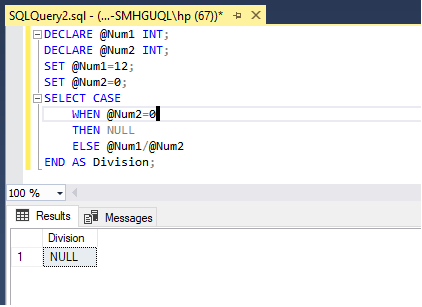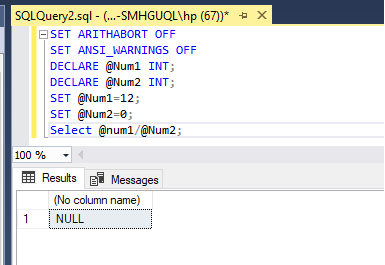# How to Avoid the “divide by Zero” Error in SQL?

In this article, we will look at how to avoid the “divide by zero” error in SQL. If we divide any number by zero, it leads to infinity, and we get an error message. We can avoid this error message using the following three methods:

• Using NULLIF() function
• Using CASE statement
• Using SET ARITHABORT OFF

We will be creating a database first to perform SQL operations.

Query:

`CREATE DATABASE Test;`

Output:Commands completed successfully show the database “Test” is created.

For all the methods we need to declare two variables that will store the values of the numerator and denominator.

```DECLARE @Num1 INT;
DECLARE @Num2 INT;```

After declaring the variable we have to set the values. Set the second variable value as zero.

```SET @Num1=12;
SET @Num2=0;```

Method 1: Using NULLIF() function

If both arguments are equal, it returns NULL. If both arguments are not equal, it returns the value of the first argument.

Syntax:

`NULLIF(exp1, exp2);`

Now we are using the NULLIF() function in the denominator with the second argument value zero.

`SELECT @Num1/NULLIF(@Num2,0) AS Division;`
• In the SQL server, if we divide any number with a NULL value its output will be NULL.
• If the first argument is zero, it means if the Num2 value is zero, then NULLIF() function returns the NULL value.
• If the first argument is not zero, then NULLIF() function returns the value of that argument. And the division takes place as regular.

Here is the complete query.

Query:

```DECLARE @Num1 INT;
DECLARE @Num2 INT;
SET @Num1=12;
SET @Num2=0;
SELECT @Num1/NULLIF(@Num2,0) AS Division;```

Output:Method 2: Using the CASE statement

The SQL CASE statement is used to check the condition and return a value. It checks the conditions until it is true and if no conditions are true it returns the value in the else part.

We have to check the value of the denominator i.e the value of the Num2 variable. If it is zero then return NULL otherwise return the regular division.

```SELECT CASE
WHEN @Num2=0
THEN NULL
ELSE @Num1/@Num2
END AS Division;```

Here is the complete query:

Query:

```DECLARE @Num1 INT;
DECLARE @Num2 INT;
SET @Num1=12;
SET @Num2=0;
SELECT CASE
WHEN @Num2=0
THEN NULL
ELSE @Num1/@Num2
END AS Division;```

Output:Method 3: SET ARITHABORT OFF

To control the behavior of queries, we can use SET methods. By default, ARITHABORT is set as ON. It terminates the query and returns an error message. If we set it OFF it will terminate and returns a NULL value.

Like ARITHBORT, we have to set ANSI_WARNINGS OFF to avoid the error message.

```SET ARITHABORT OFF;
SET ANSI_WARNINGS OFF;```

Here is the complete query:

Query:

```SET ARITHABORT OFF;
SET ANSI_WARNINGS OFF;
DECLARE @Num1 INT;
DECLARE @Num2 INT;
SET @Num1=12;
SET @Num2=0;
Select @num1/@Num2;```

Output:Unlock the Power of Placement Preparation!
Feeling lost in OS, DBMS, CN, SQL, and DSA chaos? Our Complete Interview Preparation Course is the ultimate guide to conquer placements. Trusted by over 100,000+ geeks, this course is your roadmap to interview triumph.